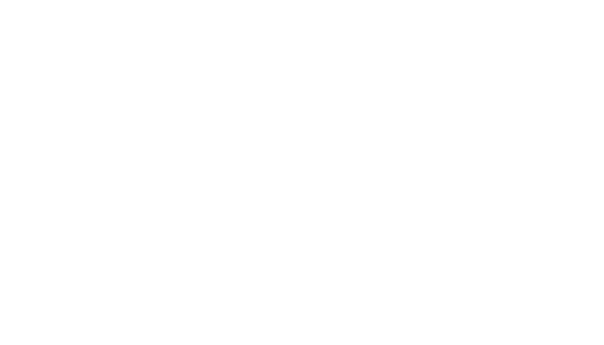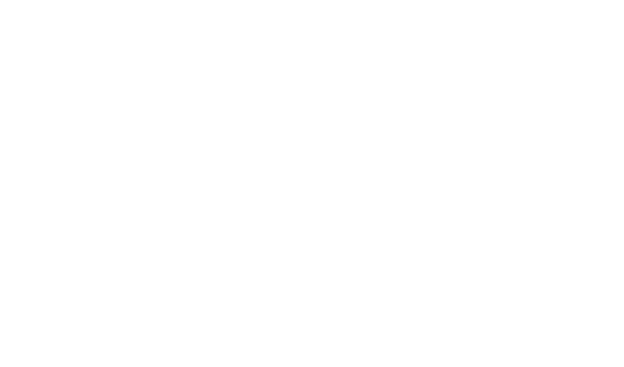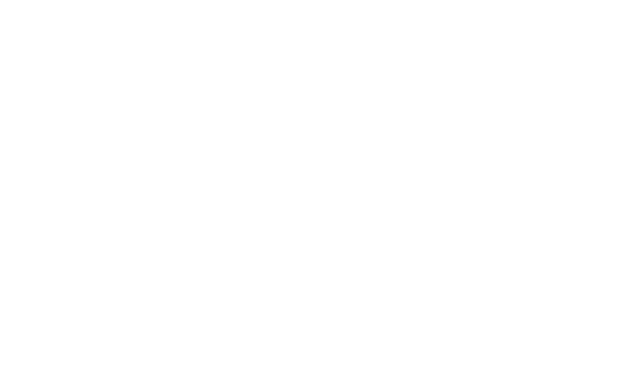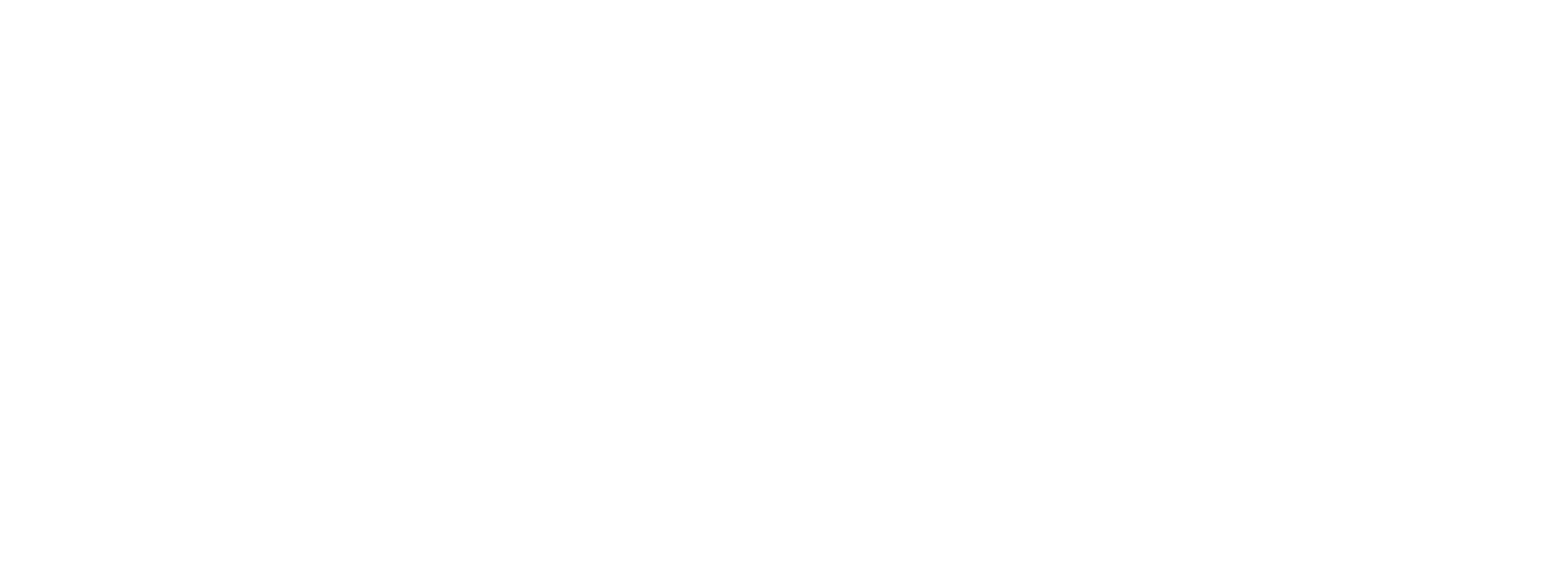# Social distancing detection using deep learning

0
1216## Introduction

In this article we are going to discuss how to Build Social Distancing Detection Tool using Deep Learning, Social distancing also called “Physical distancing” means to keep safe space distance between yourself and another human being. Is a set of non-pharmaceutical interventions or measure intended to prevent the spread of a contagious disease by maintaining a physical distance.

Social distancing should be practiced in combination with others to reduce the spread of COVID-19. As per the WHO(World Health Organization), COVID-19 has far infected almost 10 million people and claimed over 8 million lives globally. Almost 213 countries have been affected so far by this virus.

COVID-19 spread mainly among people who are in close contact (at least about 6 feet) for a prolonged period. Spread happens when an infected person coughs, sneezes, or talks, and droplets from their mouth or nose are launched into the air and land in the mouths.

Let’s build a tool that can potentially detect where each person is in real-time, and return a bounding box that turns red if the distance between two people is close.

This can be used by governments to analyze the movement of people and alert them if the situation turns serious.

## Object Detection and Tracking

Social Distancing Detection Tool using Deep Learning So, some confused object detection and image classification. Objects are everywhere, to detect the object and image classification are classified the images and very popular tasks in computer vision.

Used both of the terms in this task.

The fundamental difference between these two tasks is that image classification identifies an object in an image whereas object detection identifies the object as well as its location in an image. Here’s a classic example to understand this difference:

Object detection and tracking both terms are similar. Both tasks involve identifying the object and its location.

But, the only difference between them is the type of data that you are using. Object Detection deals with images whereas Object Tracking deals with videos.

Object Detection applied on each and every frame of a video turns into an Object Tracking problem.

As a video is a collection of fast-moving frames, Object Tracking identifies an object and its location from each and every frame of a video.

## Evolution of State-of-the-Art (SOTA) for Object Detection

Object Detection is one of the most challenging problems in computer vision. Having said that, there has been an immense improvement over the past 20 years in this field.

## Sliding Window for Object Detection

A very simple approach to building an Object Detection model is through a sliding window for Build Social Distancing Detection Tool using Deep Learning . As the name suggests, an image is divided into regions of a particular size and then every region is classified into the respective classes. This model work on CNN algorithm.

This method is really simple and efficient. But it’s a time-consuming process as it considers the huge number of regions for classification. And numbers of the matrix there mean pixel size.

Now, we will see how we can reduce the number of regions for classification in the next approach.

R-CNN for Object Detection

R-CNN stands for Region-based Convolutional Neural Network. It uses one of the external region proposal algorithms to select the region of interest (ROI).

So, now develop a less time-consuming task. Is it possible?

Yes, it is happening in a real situation, this process of extracting the regions that are likely to contain the object is known as Region Proposals.

Many Region Proposal algorithms have been proposed to select a Region of Interest (ROI). Some of the popular ones are objectness, selective search, category-independent object proposals, etc. So, R-CNN was proposed with an idea of using the exterior region proposal algorithm.

Model Workflow of R-CNN

1. Consider an image
2. Select ROI using exterior region proposal algorithm
3. For each region:
1. Pass a region to CNN
2. Extract features from CNN
3. Pass features to a classifier & regressor

The predicted regions can be overlapping and varying in size as well. So, Maximum Non Suppression is used to ignore the bounding boxes depending upon the Intersection Over Union (IOU) score:

But it consumes nearly 50 seconds for every test image during inference because of the number of forwarding passes to a CNN for feature extraction. As you can observe under the model workflow, every region proposal is passed to a CNN for feature extraction.

For example, if an image has 2000 regions of proposals, then the number of forwarding passes to the CNN is around 2000. This inevitably led to another model architecture known as Fast R-CNN.

1. Fast R-CNN for Object Detection

Widget not in any sidebars

In order to reduce the inference speed, a slight change in the R-CNN workflow was made and proposed, known as Fast R-CNN. The modification was done in the feature extraction of region proposals.

In R-CNN, feature extraction takes place for each region proposal whereas, in Fast R-CNN, feature extraction takes place only once for an original image. Then the relevant ROI features are chosen based on the location of the region proposals. These region proposals are constructed before passing an image to CNN.

Model Workflow

1. Consider an image
2. Select Regions of Interest (ROI) using exterior region proposal algorithm
3. Pass an image to the CNN
4. Extract the features of an image
5. Choose relevant ROI features using the location of ROI
6. For each ROI feature, pass features to a classifier & regressor

During inference, Fast R-CNN consumes nearly 2 seconds for each test image and is about 25 times faster than R-CNN. The reason being the change in the feature extraction of ROI. For example, if an image has a 2000 region of proposals, then the number of forwarding passes to CNN is around 1.

Can we still bring down the inference speed? Yes! It’s possible. This led to Faster R-CNN, a SOTA model for object detection tasks.

1. Faster R-CNN for Object Detection

Faster R-CNN replaces the exterior region proposal algorithm with a Region Proposal Network (RPN). RPN learns to propose the region of interest which in turn saves a lot of time and computation as compared to a Fast R-CNN.

Faster R-CNN = Fast R-CNN + RPN

Workflow model for Faster R-CNN

1. Consider an image
2. Pass an image to CNN
3. Extract the features of an image
4. Select ROI features using Region Proposal Network (RPN)
5. For each ROI feature, pass features to a classifier & regressor
1. Social Distance Tool (Tutorial)

Social Distance is the application to prevent the spread of COVID-19. This concept was launched by Andrew Ng’s.

Understanding Detectron 2

Detectron 2 is an open-source library for object detection and segmentation created by the Facebook AI Research team, popularly known as FAIR. Detectron 2 implements state of the art architectures like Faster R CNN, Mask R CNN, and RetinaNet for solving different computer vision tasks, such as:

1. Object Detection
2. Instance Segmentation
3. Keypoint Detection
4. Panoptic Segmentation

The baseline models of Faster R-CNN and Mask R-CNN are available with 3 different backbone combinations. Please refer to this Detectron-2 GitHub repository for additional details.

``````# install dependencies: (use cu101 because colab has CUDA 10.1)
!pip install cython pyyaml==5.1

# install detectron2:
!pip install detectron2==0.1.3 -f https://dl.fbaipublicfiles.com/detectron2/wheels/cu101/torch1.5/index.html

# install dependencies: (use cu101 because colab has CUDA 10.1)
!pip install cython pyyaml==5.1
!pip install -U 'git+https://github.com/cocodataset/cocoapi.git#subdirectory=PythonAPI'
import torch, torchvision
print(torch.__version__, torch.cuda.is_available())
!gcc --version
# opencv is pre-installed on colab

# install detectron2:
!pip install detectron2==0.1.3 -f https://dl.fbaipublicfiles.com/detectron2/wheels/cu101/torch1.5/index.html

Import Required Library
import detectron2
from detectron2.utils.logger import setup_logger
setup_logger()

# import some common libraries
import numpy as np
import cv2
import random
import matplotlib.pyplot as plt

# import some common detectron2 utilities
from detectron2 import model_zoo
from detectron2.engine import DefaultPredictor
from detectron2.config import get_cfg
from detectron2.utils.visualizer import Visualizer

``````%%time
!rm -r frames/*
!mkdir frames/

#specify path to video
video = "/content/sample.mp4"

#capture video
cap = cv2.VideoCapture(video)
cnt=0

# Check if video file is opened successfully
if (cap.isOpened()== False):
print("Error opening video stream or file")

while(cap.isOpened()):

# Capture frame-by-frame

if ret == True:

#save each frame to folder
cv2.imwrite('frames/'+str(cnt)+'.png', frame)
cnt=cnt+1
if(cnt==750):
break

# Break the loop
else:
break

#frame rate of a video
FPS=cap.get(cv2.CAP_PROP_FPS)
print(FPS)
``````

O/P = 25.0

Download the pre-trained model for object detection from Detectron 2’s model zoo and then the model is ready for inference:

``````cfg = get_cfg()

# add project-specific config (e.g., TensorMask) here if you're not running a model in detectron2's core library
cfg.merge_from_file(model_zoo.get_config_file("COCO-Detection/faster_rcnn_R_50_C4_3x.yaml"))
cfg.MODEL.ROI_HEADS.SCORE_THRESH_TEST = 0.9  # set threshold for this model

# Find a model from detectron2's model zoo. You can use the https://dl.fbaipublicfiles... url as well
cfg.MODEL.WEIGHTS=model_zoo.get_checkpoint_url("COCO-Detection/faster_rcnn_R_50_C4_3x.yaml")
predictor = DefaultPredictor(cfg)

Read an image and pass it to the model for predictions:

#pass to the model
#outputs = predictor(img)
print(img)

# Use `Visualizer` to draw the predictions on the image.
v = Visualizer(img[:, :, ::-1], MetadataCatalog.get(cfg.DATASETS.TRAIN), scale=1.2)
v = v.draw_instance_predictions(outputs["instances"].to("cpu"))
cv2_imshow(v.get_image()[:, :, ::-1])
``````As you can see here, multiple objects are present in an image, like a person, bicycle, and so on. We are well on our way to building the social distancing detection tool!

Next, understand the objects present in an image:

classes=outputs[‘instances’].pred_classes.cpu().numpy()

print(classes)

Have a glance at the bounding boxes of an object:

bbox=outputs[‘instances’].pred_boxes.tensor.cpu().numpy()

print(bbox)

As different objects(peoples) are present in an image, let’s identify classes and bounding boxes related to only the people:

``````#identity only persons
ind = np.where(classes==0)

#identify bounding box of only persons
person=bbox[ind]

#total no. of persons
num= len(person)
``````

Widget not in any sidebars

Now, understand the bounding box.

``````x1,y1,x2,y2 = person
print(x1,y1,x2,y2)

Try to draw a bounding box for one of the people:
_ = cv2.rectangle(img, (x1, y1), (x2, y2), (255,0,0), 2)

plt.figure(figsize=(20,10))
plt.imshow(img)
``````

Calculate the distance the bottom center for every bounding box and draw the points on the image:

``````#call the function
midpoints = [mid_point(img,person,i) for i in range(len(person))]
#visualize image
plt.figure(figsize=(20,10))
plt.imshow(img)
``````Create a function the Euclidean distance between every two points in an image:

``````points in an image:
%%time
from scipy.spatial import distance
def compute_distance(midpoints,num):
dist = np.zeros((num,num))
for i in range(num):
for j in range(i+1,num):
if i!=j:
dst = distance.euclidean(midpoints[i], midpoints[j])
dist[i][j]=dst
return dist
Now, calculate the distance between every pair of points:
dist= compute_distance(midpoints,num)
``````

Create a function that returns the closest people based on the given distance. Here, proximity distance refers to the minimum distance between two people:

``````%%time
def find_closest(dist,num,thresh):
p1=[]
p2=[]
d=[]
for i in range(num):
for j in range(i,num):
if( (i!=j) & (dist[i][j]<=thresh)):
p1.append(i)
p2.append(j)
d.append(dist[i][j])
return p1,p2,d
``````

Set the threshold for the proximity distance. I have chosen that to be 100. Let’s find the people who are within the proximity distance:

``````import pandas as pd
thresh=100
p1,p2,d=find_closest(dist,num,thresh)
df = pd.DataFrame({"p1":p1,"p2":p2,"dist":d})
df

``````
``````From the output, we can observe that 4 people come under the red zone as the distance between them is less than the proximity threshold.
Define a function to change the color of the closest people to red:
def change_2_red(img,person,p1,p2):
risky = np.unique(p1+p2)
for i in risky:
x1,y1,x2,y2 = person[i]
_ = cv2.rectangle(img, (x1, y1), (x2, y2), (255,0,0), 2)
return img
Let’s change the color of the closest people to red:
img = change_2_red(img,person,p1,p2)
plt.figure(figsize=(20,10))
plt.imshow(img)
``````Now, we have seen all procedures step by step how to apply object detection using Detectron-2, calculate the distance between every pair of people, and then finally identify the closest people. We will carry out similar steps on each and every frame of the video now:import re

names=os.listdir(‘frames/’)

names.sort(key=lambda f: int(re.sub(‘\D’, ”, f)))

def find_closest_people(name,thresh):

outputs = predictor(img)

classes=outputs[‘instances’].pred_classes.cpu().numpy()

bbox=outputs[‘instances’].pred_boxes.tensor.cpu().numpy()

ind = np.where(classes==0)

person=bbox[ind]

midpoints = [mid_point(img,person,i) for i in range(len(person))]

num = len(midpoints)

dist= compute_distance(midpoints,num)

p1,p2,d=find_closest(dist,num,thresh)

img = change_2_red(img,person,p1,p2)

cv2.imwrite(‘frames/’+name,img)

return 0

Identify the closest people in each frame and change the color to red:

from tqdm import tqdm

thresh=100

_ = [find_closest_people(names[i],thresh) for i in tqdm(range(len(names))) ]

After identifying the closest people in each frame, convert the frames back to a video. It’s done.

%%time

frames = os.listdir(‘frames/’)

frames.sort(key=lambda f: int(re.sub(‘\D’, ”, f)))

frame_array=[]

for i in range(len(frames)):

img = cv2.cvtColor(img,cv2.COLOR_BGR2RGB)

height, width, layers = img.shape

size = (width,height)

#inserting the frames into an image array

frame_array.append(img)

out = cv2.VideoWriter(‘sample_output.mp4′,cv2.VideoWriter_fourcc(*’DIVX’), 25, size)

for i in range(len(frame_array)):

# writing to a image array

out.write(frame_array[i])

out.release()

``

## Conclusion

Understanding the Build Social Distancing Detection Tool using Deep Learning using Computer Vision.I hope you have enjoyed the tutorial and found it useful.

Stay Safe Everyone.